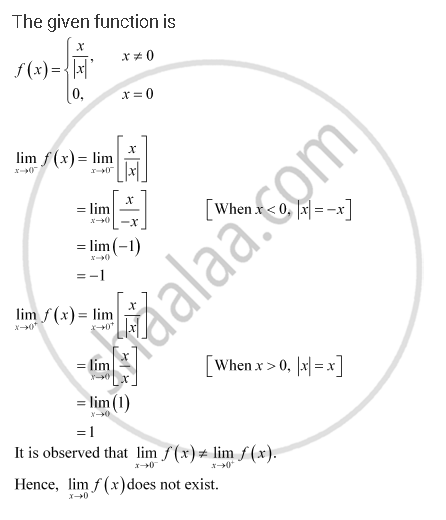CBSE (Arts) Class 11CBSE
Share

# Find Lim_(X -> 0) F(X) Where F(X) = {(X/|X|, X != 0),(0, X = 0):} - CBSE (Arts) Class 11 - Mathematics

ConceptLimits Limits of Polynomials and Rational Functions

#### Question

Find lim_(x -> 0) f(x) where f(x) = {(x/|x|, x != 0),(0, x = 0):}

#### SolutionIs there an error in this question or solution?

#### APPEARS IN

NCERT Solution for Mathematics Textbook for Class 11 (2018 to Current)
Chapter 13: Limits and Derivatives
Q: 26 | Page no. 302

#### Video TutorialsVIEW ALL 

Solution Find Lim_(X -> 0) F(X) Where F(X) = {(X/|X|, X != 0),(0, X = 0):} Concept: Limits - Limits of Polynomials and Rational Functions.
S